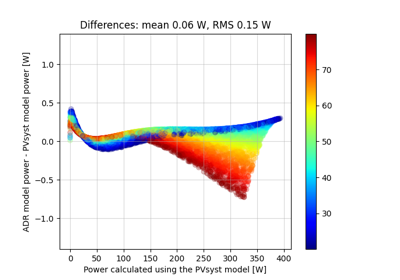# pvlib.pvsystem.calcparams_pvsyst#

pvlib.pvsystem.calcparams_pvsyst(effective_irradiance, temp_cell, alpha_sc, gamma_ref, mu_gamma, I_L_ref, I_o_ref, R_sh_ref, R_sh_0, R_s, cells_in_series, R_sh_exp=5.5, EgRef=1.121, irrad_ref=1000, temp_ref=25)[source]#

Calculates five parameter values for the single diode equation at effective irradiance and cell temperature using the PVsyst v6 model. The PVsyst v6 model is described in 1, 2, 3. The five values returned by calcparams_pvsyst can be used by singlediode to calculate an IV curve.

Parameters
• effective_irradiance (numeric) – The irradiance (W/m2) that is converted to photocurrent.

• temp_cell (numeric) – The average cell temperature of cells within a module in C.

• alpha_sc (float) – The short-circuit current temperature coefficient of the module in units of A/C.

• gamma_ref (float) – The diode ideality factor

• mu_gamma (float) – The temperature coefficient for the diode ideality factor, 1/K

• I_L_ref (float) – The light-generated current (or photocurrent) at reference conditions, in amperes.

• I_o_ref (float) – The dark or diode reverse saturation current at reference conditions, in amperes.

• R_sh_ref (float) – The shunt resistance at reference conditions, in ohms.

• R_sh_0 (float) – The shunt resistance at zero irradiance conditions, in ohms.

• R_s (float) – The series resistance at reference conditions, in ohms.

• cells_in_series (integer) – The number of cells connected in series.

• R_sh_exp (float) – The exponent in the equation for shunt resistance, unitless. Defaults to 5.5.

• EgRef (float) – The energy bandgap at reference temperature in units of eV. 1.121 eV for crystalline silicon. EgRef must be >0.

• temp_ref (float (optional, default=25)) – Reference cell temperature in C.

Returns

• Tuple of the following results

• photocurrent (numeric) – Light-generated current in amperes

• saturation_current (numeric) – Diode saturation current in amperes

• resistance_series (numeric) – Series resistance in ohms

• resistance_shunt (numeric) – Shunt resistance in ohms

• nNsVth (numeric) – The product of the usual diode ideality factor (n, unitless), number of cells in series (Ns), and cell thermal voltage at specified effective irradiance and cell temperature.

References

1

K. Sauer, T. Roessler, C. W. Hansen, Modeling the Irradiance and Temperature Dependence of Photovoltaic Modules in PVsyst, IEEE Journal of Photovoltaics v5(1), January 2015.

2

A. Mermoud, PV modules modelling, Presentation at the 2nd PV Performance Modeling Workshop, Santa Clara, CA, May 2013

3

A. Mermoud, T. Lejeune, Performance Assessment of a Simulation Model for PV modules of any available technology, 25th European Photovoltaic Solar Energy Conference, Valencia, Spain, Sept. 2010

## Examples using `pvlib.pvsystem.calcparams_pvsyst`#Fast simulation using the ADR efficiency model starting from PVsyst parameters

Fast simulation using the ADR efficiency model starting from PVsyst parameters